Question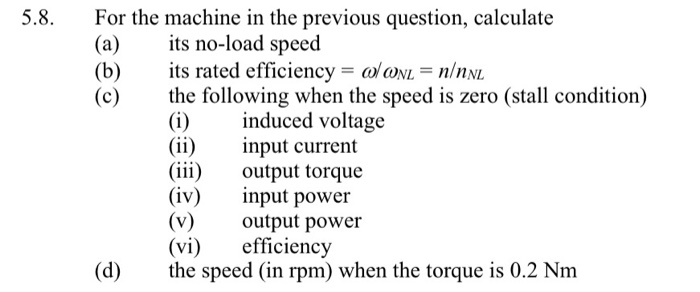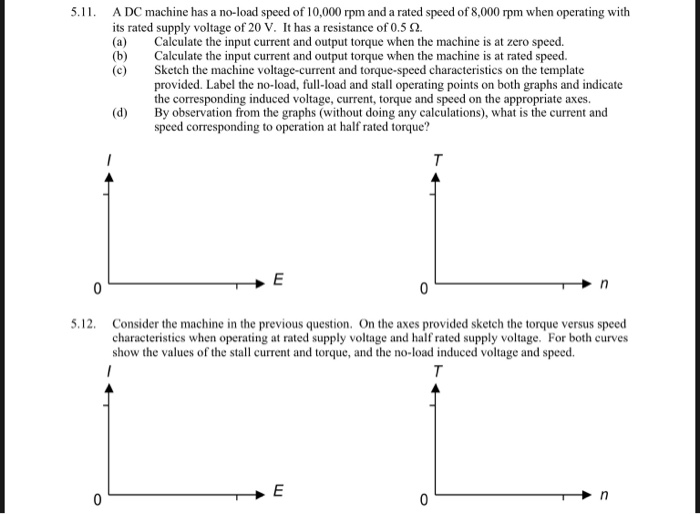Q5.8：The specifications of machine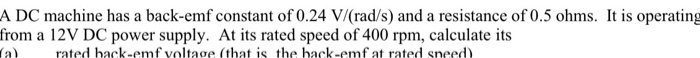5.8. For the machine in the previous question, calculate (a) its no-load speed (b)its rated efficiency-olcoxL-n/nN (c) the following when the speed is zero (stall condition) (i)induced voltage (ii) nput current (ii) output torque (iv) ut power (v)output power (vi) efficiency (d) he speed (in rpm) when the torque is 0.2 Nm
5 A DC machine has a no-load speed of 10,000 rpm and a rated speed of 8,000 rpm when operating with its rated supply voltage of 20 V. It has a resistance of 0.52. (a) Calculate the input current and output torque when the machine is at zero speed. (b) Calculate the input current and output torque when the machine is at rated speed (c) Sketch the machine voltage-current and torque-speed characteristics on the template provided. Label the no-load, full-load and stall operating points on both graphs and indicate the corresponding induced voltage, current, torque and speed on the appropriate axes. By observation from the graphs (without doing any calculations), what is the current and speed corresponding to operation at half rated torque? (d) 5.12. Consider the machine in the previous question. On the axes provided sketch the torque versus speed characteristies when operating at rated supply voltage and half rated supply voltage. For both curves show the values of the stall current and torque, and the no-load induced voltage and speed
A DC machine has a back-emf constant of 0.24 V/(rad/s) and a resistance of 0.5 ohms. It is operating from a 12V DC power supply. At its rated speed of 400 rpm, calculate its rated hack-emf voltage (that is the hack-emf at rated sneed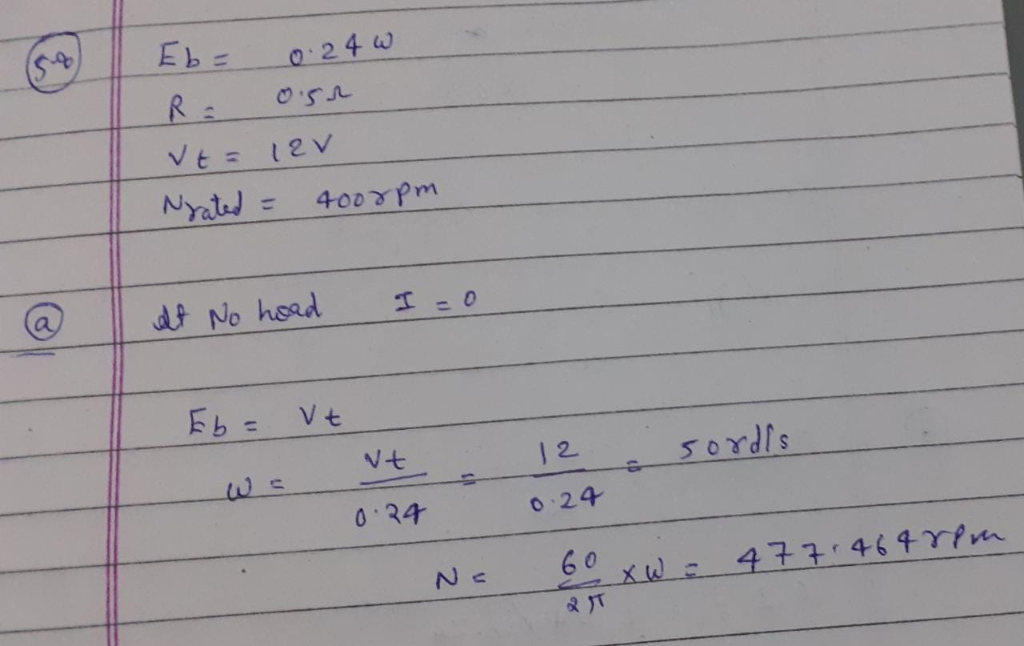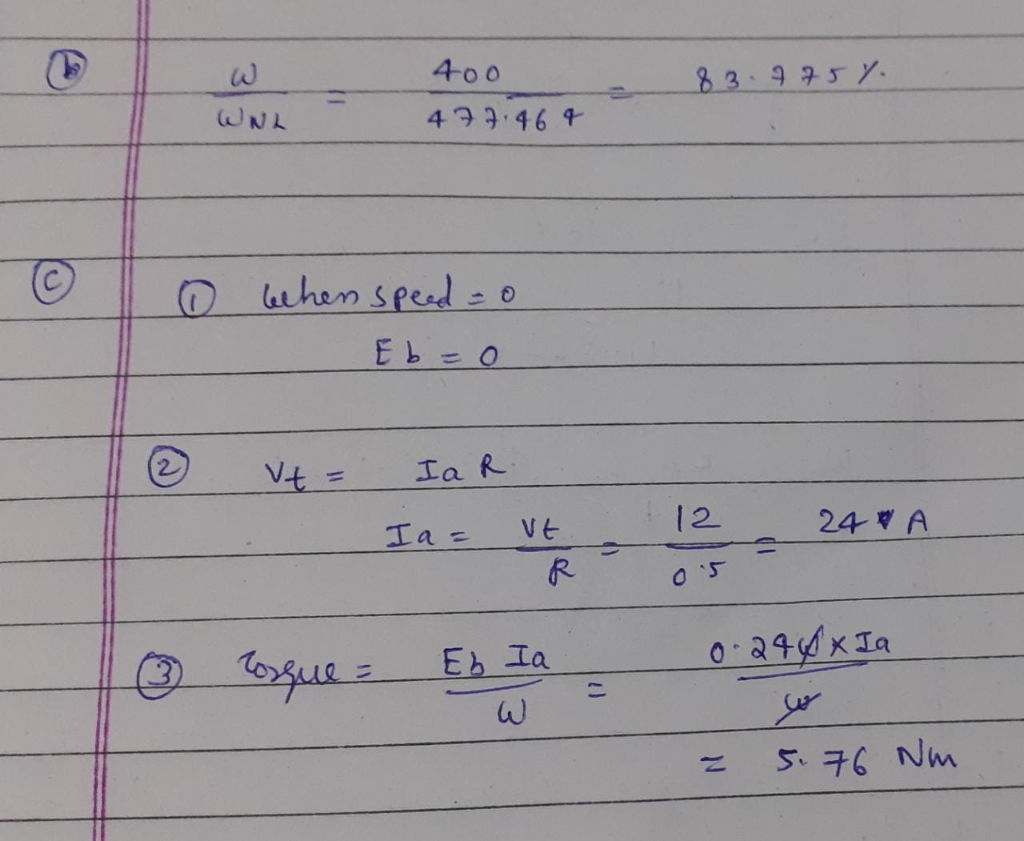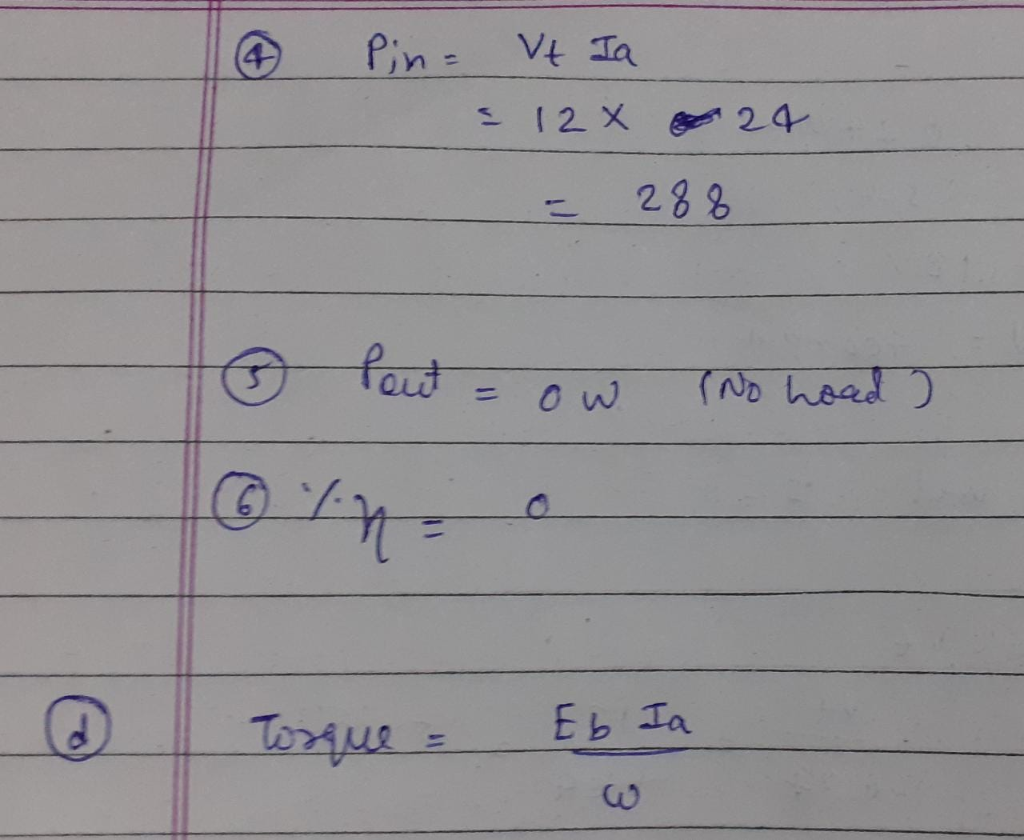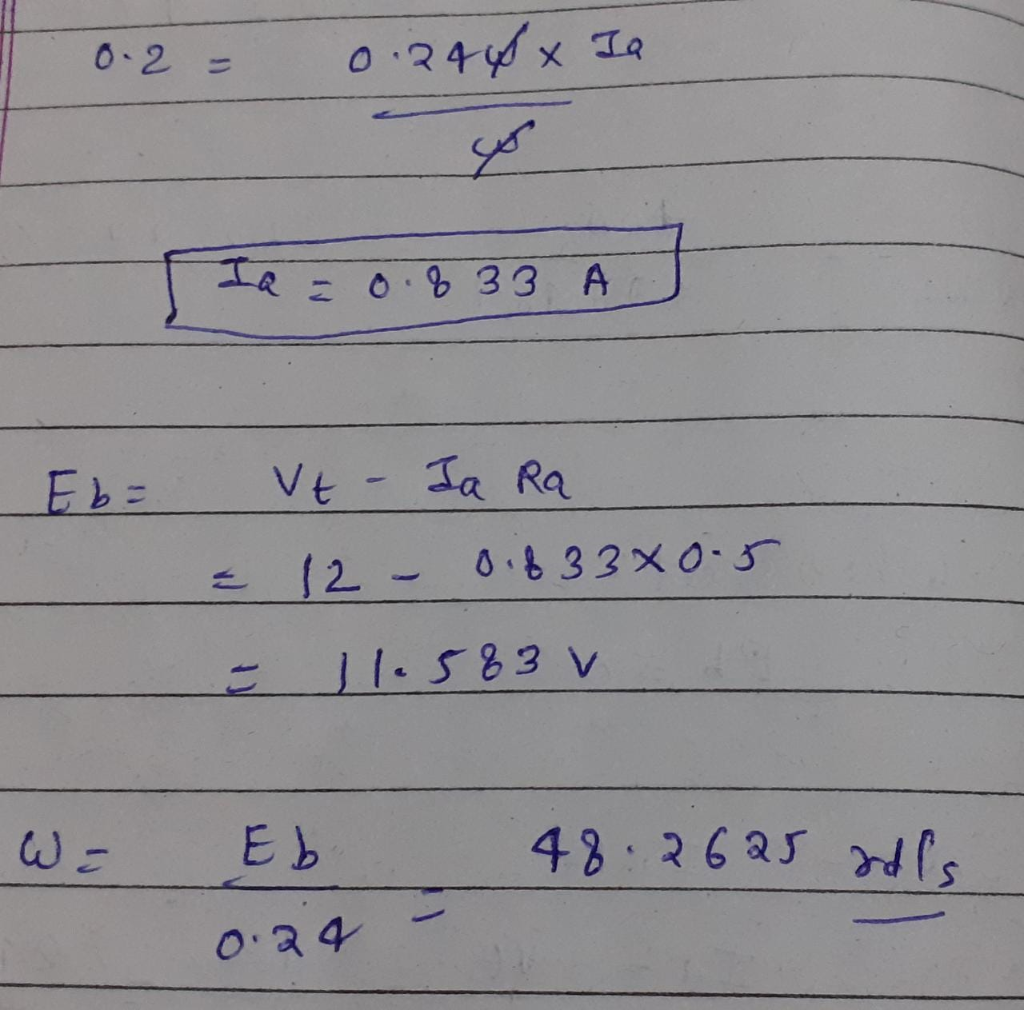#### Earn Coins

Coins can be redeemed for fabulous gifts.

Similar Homework Help Questions
• ### 5.7. A DC machine has a back-emf constant of 0.24 V/(rad/s) and a resistance of 0.5...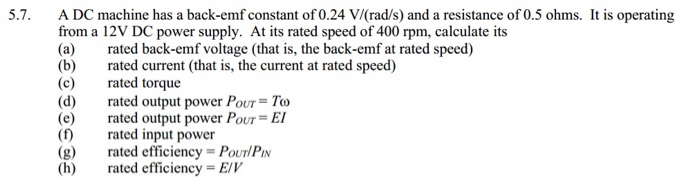5.7. A DC machine has a back-emf constant of 0.24 V/(rad/s) and a resistance of 0.5 ohms. It is operating from a 12V DC power supply. At its rated speed of 400 rpm, calculate its (a) rated back-emf voltage (that is, the back-emf at rated speed) (b)rated current (that is, the current at rated speed) (c) rated torque (d) rated output power POUT= To (e) rated output power POUT = EI (f) rated input power (g) rated efficiency-PouTlPi (h) rated...

• ### Q1b) DC Machines [ 6 marks ] You are given a 24 V DC motor. When...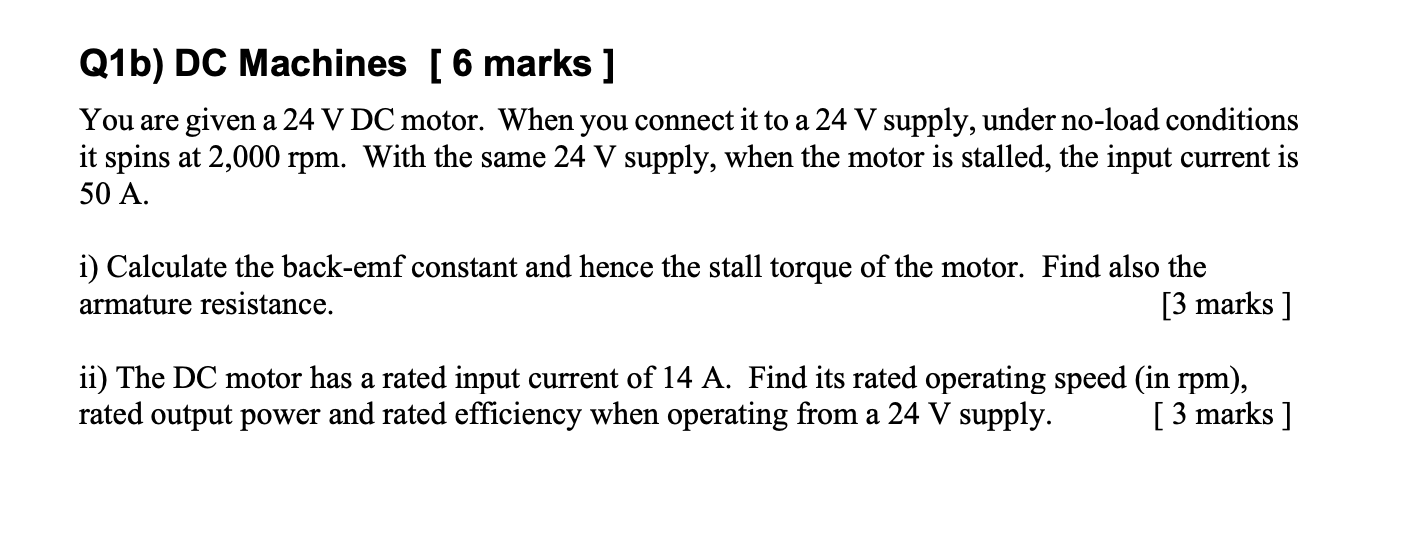Q1b) DC Machines [ 6 marks ] You are given a 24 V DC motor. When you connect it to a 24 V supply, under no-load conditions it spins at 2,000 rpm. With the same 24 V supply, when the motor is stalled, the input current is 50 A. i) Calculate the back-emf constant and hence the stall torque of the motor. Find also the armature resistance. [3 marks ] ii) The DC motor has a rated input current of...

• ### A 12-pole, brushless, DC motor has a no-load speed of 5000rpm and a stall torque of 50 in-oz when operating at rated voltage of 60V. Testing shows that no-load speed and stall torque vary linearly wi...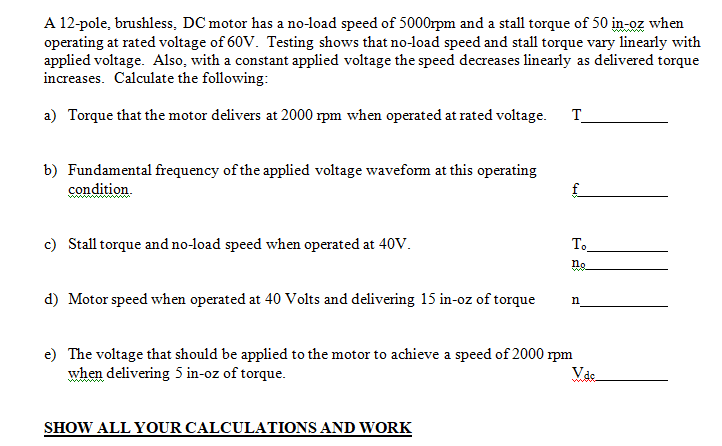A 12-pole, brushless, DC motor has a no-load speed of 5000rpm and a stall torque of 50 in-oz when operating at rated voltage of 60V. Testing shows that no-load speed and stall torque vary linearly with applied voltage. Also, with a constant applied voltage the speed decreases linearly as delivered torque increases. Calculate the following: Torque that the motor delivers at 2000 rpm when operated at rated voltage. T a) b) Fundamental frequency of the applied voltage waveform at this...

• ### A separated excited dc machine is delivering 50% rated torque at 20% rated speed. Find the...

A separated excited dc machine is delivering 50% rated torque at 20% rated speed. Find the efficiency of the motor at this operating point. The details of the machine are as follows: 1500 kW(rated output power), 600V(rated voltage), 2650A (rated current) 600rpm (rated speed), brush voltage drop = 2V, Filed power input = 50 kW, Ra=3.645 mOhm, La=0.1 mH, Machine frictional torque coefficient = 15 Nm/rad/s. Field current is constant and armature voltage is variable.

• ### A 230 V shunt direct-current machine on no-load has a speed of 900 rpm and a...

A 230 V shunt direct-current machine on no-load has a speed of 900 rpm and a supply current of 2.5 A. The machine parameters are: • Field resistance: 230 Ω • Armature resistance: 0.3 Ω • Voltage drop/brush: 1.0 V (current independent) a) Calculate the electromagnetic torque, speed, the output power and the overall efficiency when the supply current is 35 A, assuming that the friction and wind-age torque is constant. (16 points) b) The machine operates now as generator...

• ### For a PMDC motor powered by a 12 V DC source, the no-load speed and stall...

For a PMDC motor powered by a 12 V DC source, the no-load speed and stall torque are 3800 RPM and 30 mNm, respectively. If the motor is running at 2500 RPM, find a) Shaft torque (Tshaft), b) Rotor current (Im), c) Back emf (Eb), d) Electrical power consumed, e) Shaft power, and f) Power loss in rotor coil.

• ### need the answer for the question no. 1 ENGR 113 Homework DC Motor Objectives Plot the...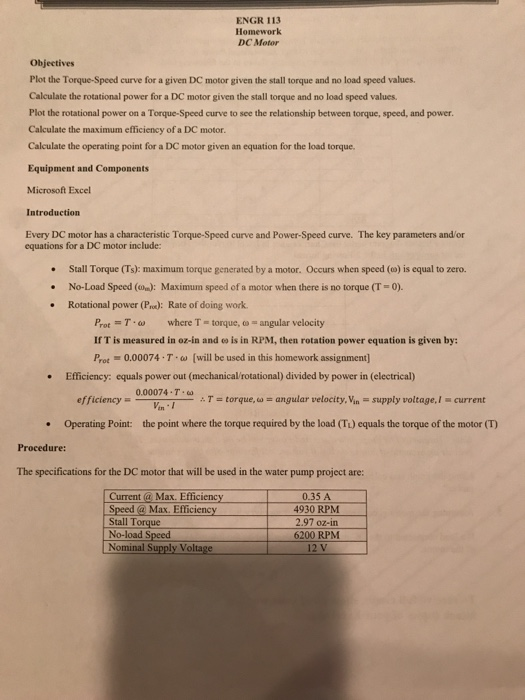need the answer for the question no. 1 ENGR 113 Homework DC Motor Objectives Plot the Torque-Speed curve for a given DC motor given the stall torque and no load speed values Calculate the rotational power for a DC motor given the stall torque and no load speed values Plot the rotational power on a Torque-Speed curve to see the relationship between torque, speed, and power Calculate the maximum efficiency of a DC motor. Calculate the operating point for a...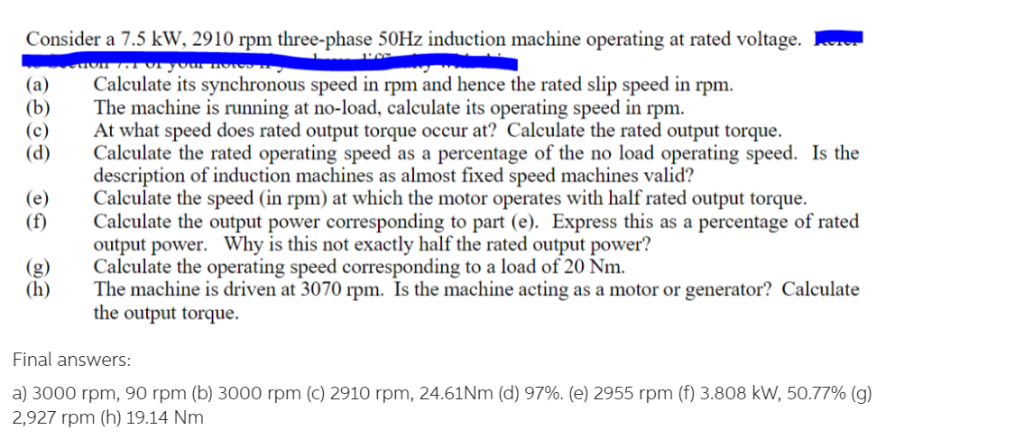Please show all steps with clear hand-writing. Final answers are provided in the bottom and must match with your answers. Consider a 7.5 kW, 2910 rpm three-phase 50Hz induction machine operating at rated voltage. (a) Calculate its synchronous speed in rpm and hence the rated slip speed in rpm. (b)The machine is running at no-load, calculate its operating speed in rpm (c) At what speed does rated output torque occur at? Calculate the rated output torque. (d) Calculate the rated...

• ### An eight-pole, 25 kW, 120 V DC generator has a duplex lap-wound armature, which has 64 coils with 16 turns per coil. Its rated speed is 2400 rpm (HELP)Question:  An eight-pole, 25 kW, 120 V DC generator has a duplex lap-wound armature, which has 64 coils with 16 turns per coil. Its rated speed is 2400 rpm.(a)   Define a duplex lap-wound DC armature.                                                      (b)   How much flux per pole is required to produce the rated voltage in this generator at no load conditions?                                                                                        (c)   What is the induced torque in this machine at rated load?                            (d)   Determine path current in the armature of this generator at the rated load? ...

• ### A 230 V shunt direct-current machine on no-load has a speed of 900 rpm and a supply current of 2.5 A. The machine parameters are: • Field resistance: 230 Ω • Armature resistance: 0.3 Ω • Voltage drop/...

A 230 V shunt direct-current machine on no-load has a speed of 900 rpm and a supply current of 2.5 A. The machine parameters are: • Field resistance: 230 Ω • Armature resistance: 0.3 Ω • Voltage drop/brush: 1.0 V (current independent) a) Calculate the electromagnetic torque, speed, the output power and the overall efficiency when the supply current is 35 A, assuming that the friction and wind-age torque is constant. (16 points) b) The machine operates now as generator...